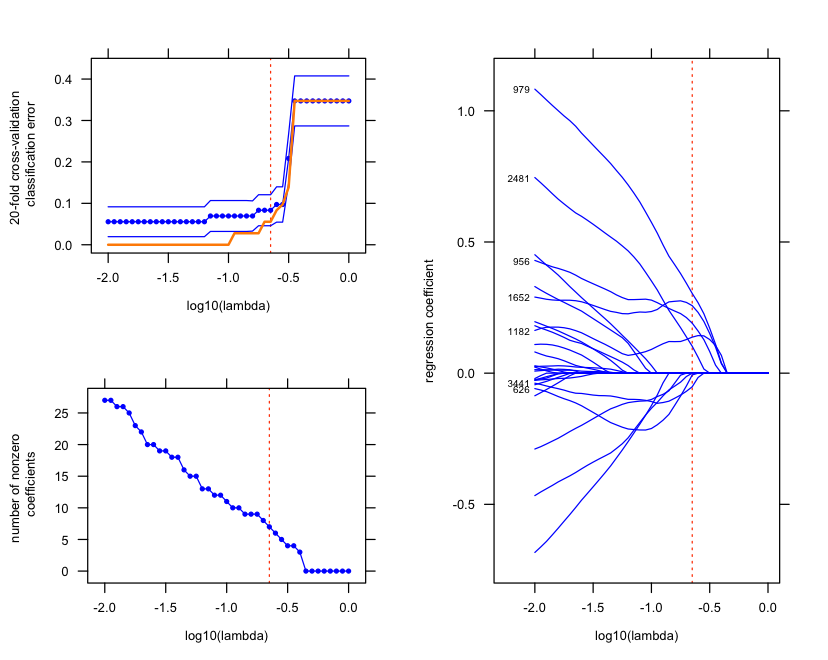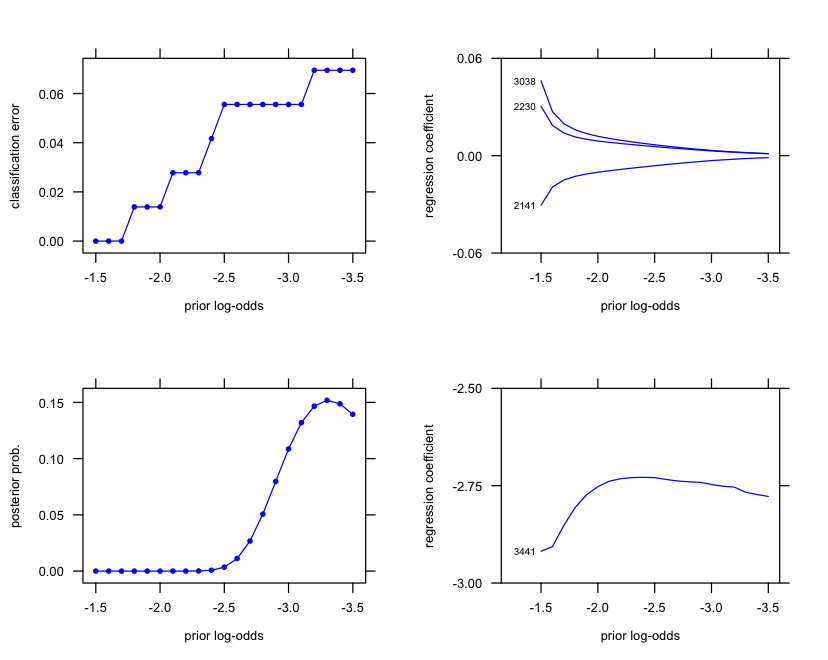This vignette demonstrates application of glmnet and varbvs to the Leukemia data set. The main aim of this script is to illustrate some of the different properties of Bayesian variable selection and penalized sparse regression (as implemented by varbvs and glmnet, respectively). This script also reproduces the results and graphs presented in the first example of a forthcoming paper (Carbonetto et al, 2016).

We use the preprocessed data of Dettling (2004) retrieved from the supplementary materials accompanying Friedman et al (2010). The data are represented as a 72 x 3,571 matrix of gene expression values (variable `X`), and a vector of 72 binary disease outcomes (variable `y`).

### References

Carbonetto, P., et al (2016) Fast variable selection for genome-wide association studies and other large-scale regression applications. Forthcoming.

Dettling, M. (2004). BagBoosting for tumor classification with gene expression data. Bioinformatics 20, 3583–3593.

Friedman, J., Hastie, T., Tibshirani, R. (2010) Regularization paths for generalized linear models via coordinate descent. Journal of Statistical Software 33, 1–22.

## Vignette parameters

Begin by loading a few packages into the R environment.

``````library(lattice)
library(latticeExtra)
library(glmnet)
library(varbvs)
``````

Next, specify settings that control the data analyses.

``````# glmnet settings.
nfolds <- 20                   # Number of cross-validation folds.
alpha  <- 0.95                 # Elastic net mixing parameter.
lambda <- 10^(seq(-2,0,0.05))  # Lambda sequence.

# varbvs settings.
logodds <- seq(-3.5,-1.5,0.1)  # Candidate prior log-odds settings.
sa      <- 1                   # Prior variance of coefficients.
``````

## Load the Leukemia data

Also set the random number generator seed.

``````data(leukemia)
X <- leukemia\$x
y <- leukemia\$y
set.seed(1)
``````

## Fit elastic net model to data

Here, we also run 20-fold cross-validation to select the largest setting of the L1-penalty strength (lambda) that is within 1 standard error of the minimum classification error.

``````
# This is the model fitting step.
r <- system.time(fit.glmnet <-
glmnet(X,y,family = "binomial",lambda = lambda,alpha = alpha))
cat(sprintf("Model fitting took %0.2f seconds.\n",r["elapsed"]))
# Model fitting took 0.70 seconds.

# This is the cross-validation step.
r <- system.time(out.cv.glmnet <-
cv.glmnet(X,y,family = "binomial",type.measure = "class",
alpha = alpha,nfolds = nfolds,lambda = lambda))
lambda <- out.cv.glmnet\$lambda
cat(sprintf("Cross-validation took %0.2f seconds.\n",r["elapsed"]))
# Cross-validation took 3.65 seconds.

# Choose the largest value of lambda that is within 1 standard error
# of the smallest misclassification error.
lambda.opt <- out.cv.glmnet\$lambda.1se
``````

## Evaluate the glmnet predictions

Compute estimates of the disease outcome using the fitted model, and compare against the observed values.

``````cat("classification results with lambda = ",lambda.opt,":\n",sep="")
y.glmnet <- c(predict(fit.glmnet,X,s = lambda.opt,type = "class"))
print(table(true = factor(y),pred = factor(y.glmnet)))
# classification results with lambda = 0.2238721:
#     pred
# true  0  1
#    0 47  0
#    1  4 21
``````

## Visualize results of glmnet analysis

The first plot shows the evolution of regression coefficients at different settings of lambda. (Note that the intercept is not shown.) Only the curves for the variables that are selected at the optimal setting of lambda (“lambda.opt”“) are labeled.

The second plot shows the classification error at different settings of lambda.

The third plot shows the number of nonzero regression coefficients at different settings of lambda.

``````trellis.par.set(par.xlab.text = list(cex = 0.65),
par.ylab.text = list(cex = 0.65),
axis.text     = list(cex = 0.65))

# Plot regression coefficients.
vars <- setdiff(which(rowSums(abs(coef(fit.glmnet))) > 0),1)
n    <- length(vars)
b    <- as.matrix(t(coef(fit.glmnet)[vars,]))
i    <- coef(fit.glmnet,s = lambda.opt)
i    <- rownames(i)[which(i != 0)]
i    <- i[-1]
vars.opt <- colnames(b)
vars.opt[!is.element(vars.opt,i)] <- ""
vars.opt <- substring(vars.opt,2)
r    <- xyplot(y ~ x,data.frame(x = log10(lambda),y = b[,1]),type = "l",
col = "blue",xlab = "log10(lambda)",
ylab = "regression coefficient",
scales = list(x = list(limits = c(-2.35,0.1)),
y = list(limits = c(-0.8,1.2))),
panel = function(x, y, ...) {
panel.xyplot(x,y,...);
panel.abline(v = log10(lambda.opt),col = "orangered",
lwd = 1,lty = "dotted");
ltext(x = -2,y = b[nrow(b),],labels = vars.opt,pos = 2,
offset = 0.5,cex = 0.5)
})
for (i in 2:n)
r <- r + as.layer(xyplot(y ~ x,data.frame(x = log10(lambda),y = b[,i]),
type = "l",col = "blue"))
print(r,split = c(2,1,2,1),more = TRUE)

# Plot classification error.
Y       <- predict(fit.glmnet,X,type = "class")
mode(Y) <- "numeric"
print(with(out.cv.glmnet,
xyplot(y ~ x,data.frame(x = log10(lambda),y = cvm),type = "l",
col = "blue",xlab = "log10(lambda)",
ylab = "20-fold cross-validation \n classification error",
scales = list(y = list(limits = c(-0.02,0.45))),
panel = function(x, y, ...) {
panel.xyplot(x,y,...)
panel.abline(v = log10(lambda.opt),col = "orangered",
lwd = 1,lty = "dotted")
}) +
as.layer(xyplot(y ~ x,data.frame(x = log10(lambda),y = cvm),
pch = 20,cex = 0.6,col = "blue")) +
as.layer(xyplot(y ~ x,data.frame(x = log10(lambda),y = cvup),
type = "l",col = "blue",lty = "solid")) +
as.layer(xyplot(y ~ x,data.frame(x = log10(lambda),y = cvlo),
type = "l",col = "blue",lty = "solid")) +
as.layer(xyplot(y ~ x,data.frame(x = log10(lambda),
y = colMeans(abs(Y - y))),
type = "l",col = "darkorange",lwd = 2,
lty = "solid"))),
split = c(1,1,2,2),more = TRUE)

# Plot number of nonzero regression coefficients.
print(with(out.cv.glmnet,
xyplot(y ~ x,data.frame(x = log10(lambda),y = nzero),type = "l",
col = "blue",xlab = "log10(lambda)",
ylab = "number of nonzero \n coefficients",
panel = function(x, y, ...) {
panel.xyplot(x,y,...)
panel.abline(v = log10(lambda.opt),col = "orangered",
lwd = 1,lty = "dotted")
}) +
as.layer(xyplot(y ~ x,data.frame(x = log10(lambda),y = nzero),
pch = 20,cex = 0.6,col = "blue"))),
split = c(1,2,2,2),more = FALSE)
``````## Fit variational approximation to posterior

Fit the fully-factorized variational approximation to the posterior distribution of the coefficients for a logistic regression model of the binary outcome (the type of leukemia), with spike-and-slab priors on the coefficients.

``````r <- system.time(fit.varbvs <-
varbvs(X,NULL,y,"binomial",logodds = logodds,sa = sa,verbose = FALSE))
cat(sprintf("Model fitting took %0.2f seconds.\n",r["elapsed"]))
# Model fitting took 35.74 seconds.
``````

Compute the normalized importance weights and the posterior inclusion probabilities averaged over the hyperparameter settings.

``````w   <- normalizelogweights(fit.varbvs\$logw)
pip <- c(fit.varbvs\$alpha %*% w)
``````

## Evaluate the varbvs predictions

Compute estimates of the disease outcome using the fitted model, and compare against the observed values.

``````y.varbvs <- predict(fit.varbvs,X)
print(table(true = factor(y),pred = factor(y.varbvs)))
#     pred
# true  0  1
#    0 45  2
#    1  3 22
``````

## Visualize results of varbvs analysis

The first plot shows the classification error at each setting of the prior log-odds.

The second plot shows the evolution of the posterior mean regression coefficients (the beta's) at different settings of the prior log-odds, for the top 6 variables ranked by posterior inclusion probability (averaged over settings of the hyperparameters).

The top-ranked variable (by posterior inclusion probability) has a much larger coefficient than all the others, so it is shown in a separate plot.

The third plot shows the (approximate) probability density of the prior log-odds parameter.

``````trellis.par.set(par.xlab.text = list(cex = 0.65),
par.ylab.text = list(cex = 0.65),
axis.text     = list(cex = 0.65))

# Plot classification error.
m   <- length(logodds)
err <- rep(0,m)
for (i in 1:m) {
r      <- logodds[i]
ypred  <- predict(subset(fit.varbvs,logodds == r),X)
err[i] <- mean(y != ypred)
}
print(xyplot(y ~ x,data.frame(x = logodds,y = err),type = "l",
col = "blue",xlab = "prior log-odds",
ylab = "classification error",
scales = list(x = list(limits = c(-1.4,-3.6)))) +
as.layer(xyplot(y ~ x,data.frame(x = logodds,y = err),
col = "blue",pch = 20,cex = 0.65)),
split = c(1,1,2,2),more = TRUE)

# Plot regression coefficients.
n     <- 4
m     <- length(logodds)
vars  <- order(pip,decreasing = TRUE)[1:n]
mu    <- t(fit.varbvs\$alpha[vars,] * fit.varbvs\$mu[vars,])
print(xyplot(y ~ x,data.frame(x = logodds,y = (mu[,1])),
type = "l",col = "blue",xlab = "prior log-odds",
ylab = "regression coefficient",
scales = list(x = list(limits = c(-1.15,-3.6)),
y = list(limits=c(-3,-2.5),at=seq(-3,-2.5,0.25))),
panel = function(x, y, ...) {
panel.xyplot(x,y,...);
ltext(x = -1.5,y = mu[m,1],
labels = vars,pos = 2,
offset = 0.5,cex = 0.5)
}),
split = c(2,2,2,2),more = TRUE)

r    <- xyplot(y ~ x,data.frame(x = logodds,y = (mu[,2])),
type = "l",col = "blue",xlab = "prior log-odds",
ylab = "regression coefficient",
scales = list(x = list(limits = c(-1.15,-3.6)),
y = list(limits = c(-0.06,0.06),
at = c(-0.06,0,0.06))),
panel = function(x, y, ...) {
panel.xyplot(x,y,...);
ltext(x = -1.5,y = mu[m,],
labels = vars,pos = 2,
offset = 0.5,cex = 0.5)
})
for (i in 3:n) {
r <- r + as.layer(xyplot(y ~ x,
data.frame(x = logodds,y = mu[,i]),
type = "l",col = "blue"))
}
print(r,split = c(2,1,2,2),more = TRUE)

# Plot density of "prior log-odds"" hyperparameter.
names(w) <- logodds
print(xyplot(y ~ x,data.frame(x = logodds,y = w),type = "l",col = "blue",
xlab = "prior log-odds",ylab = "posterior prob.",
scales = list(x = list(limits = c(-1.4,-3.6)))) +
as.layer(xyplot(y ~ x,data.frame(x = logodds,y = w),
col = "blue",pch = 20,cex = 0.65)),
split = c(1,2,2,2),more = FALSE)
``````